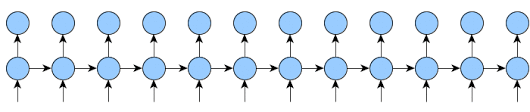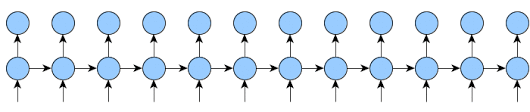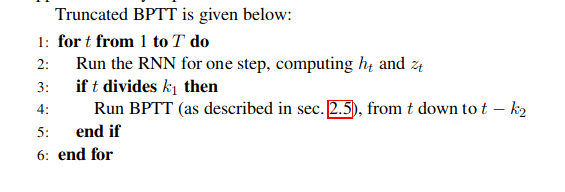# Implementing Truncated Backpropagation Through Time

(Adrián Javaloy) #1

Hello,
I’m implementing a recursive network that is going to be trained with very long sequences. I had memory problems when training because of that excessive length and I decided to use a truncated-BPTT algorithm to train it as described here, that is,

`every k1 steps backpropagate taking k2 back steps`

checking some examples I could easily write the case when `k1 = k2`. However, I haven’t been able to implement the general case yet.

My first idea was to freeze the gradient graph after the first `k2` steps and keep changing the `Variables` referenced there. Later, I saw that the graph doesn’t directly reference earlier Variables, instead it directly incorporates the gradient graphs from that `Variables`. So I thought about looking for that copied subgraphs and substituting them by a `Variable` reference, but I found out that the gradient graph is unwritable and immutable.

The only idea I have left is to recompute the calculations in the overlaps between backpropagations. That’s going to work, but I’d really love to avoid recomputing things.

Any idea on how to implement this efficiently?

2 Likes
How to train RNN with infinite sequences?
(Alban D) #2

Just to be sure before I write something long that is not what you asked:

• You have an `nn.Module` (lets call it `one_step_module`) that does one step given a current state, an input and produce an output
• You have another `nn.Module` (lets call it `loss_module`) that given the output and a target gives you the loss for this output.

And in pseudo code, what you want is:

``````state = init_state
for i, (inp, target) in enumerate(my_very_long_sequence_of_inputs):
output, state = one_step_module(inp, state)
if (i+1)%k1 == 0:
loss = loss_module(output, target)
# You want the function below
loss.backward_only_k2_last_calls_to_one_step_module()
``````
(Adrián Javaloy) #3

That’s pretty much what I want to do, indeed. So far I just detach the variables inside that if statement, so I achieve the effect of having `k1 = k2`.

Maybe I should have put some pseudocode from the very beginning. Sorry about that.

(Alban D) #4

Here is an implementation that will work for any k1 and k2 and will reduce memory usage as much as possible.
If k2 is not huge and the `one_step_module` is relatively big, the slowdown of doing multiple backward should be negligible.

The code is not super clean and has been tested only against current master branch (where Variable and Tensor are merged) so you might need slight modifications if you use 0.3.
Hope this helps.

``````
class TBPTT():
def __init__(self, one_step_module, loss_module, k1, k2, optimizer):
self.one_step_module = one_step_module
self.loss_module = loss_module
self.k1 = k1
self.k2 = k2
self.retain_graph = k1 < k2
# You can also remove all the optimizer code here, and the
# train function will just accumulate all the gradients in
# one_step_module parameters
self.optimizer = optimizer

def train(self, input_sequence, init_state):
states = [(None, init_state)]
for j, (inp, target) in enumerate(input_sequence):

state = states[-1].detach()
state.requires_grad=True
output, new_state = self.one_step_module(inp, state)
states.append((state, new_state))

while len(states) > self.k2:
# Delete stuff that is too old
del states

if (j+1)%self.k1 == 0:
loss = self.loss_module(output, target)

optimizer.zero_grad()
# backprop last module (keep graph only if they ever overlap)
start = time.time()
loss.backward(retain_graph=self.retain_graph)
for i in range(self.k2-1):
# if we get all the way back to the "init_state", stop
if states[-i-2] is None:
break
curr_grad = states[-i-1].grad
states[-i-2].backward(curr_grad, retain_graph=self.retain_graph)
print("bw: {}".format(time.time()-start))
optimizer.step()

seq_len = 20
layer_size = 50

idx = 0

class MyMod(nn.Module):
def __init__(self):
super(MyMod, self).__init__()
self.lin = nn.Linear(2*layer_size, 2*layer_size)

def forward(self, inp, state):
global idx
full_out = self.lin(torch.cat([inp, state], 1))
# out, new_state = full_out.chunk(2, dim=1)
out = full_out.narrow(1, 0, layer_size)
new_state = full_out.narrow(1, layer_size, layer_size)
def get_pr(idx_val):
def pr(*args):
print("doing backward {}".format(idx_val))
return pr
new_state.register_hook(get_pr(idx))
out.register_hook(get_pr(idx))
print("doing fw {}".format(idx))
idx += 1
return out, new_state

one_step_module = MyMod()
loss_module = nn.MSELoss()
input_sequence = [(torch.rand(200, layer_size), torch.rand(200, layer_size))] * seq_len

optimizer = torch.optim.SGD(one_step_module.parameters(), lr=1e-3)

runner = TBPTT(one_step_module, loss_module, 5, 7, optimizer)

runner.train(input_sequence, torch.zeros(200, layer_size))
print("done")
``````
6 Likes
Delete variable from the graph
(Adrián Javaloy) #5

So the idea of your code is to isolate the variables in each time-step and every `k1` steps “rewire” the last `k2` states, right? I like it, I should’ve found it out myself to be fair.

And I agree that as long as `k2 - k1` isn’t too big the overhead should be negligible.

Thanks for your help @albanD!2 Likes
(Alban D) #6

Yes, that is exactly the idea !

Happy it helps#7

I have a very similar problem where I am trying to unroll a recurrent neural network, in my case I don’t use truncated backprop but just BPTT. The network takes as input the previous output and my training code looks like:

``````for epoch in range(1, args.epochs + 1):
model.train()
epoch_loss = 0
for i, sequence in enumerate(training_data_loader):
optimizer.zero_grad()
loss = 0

output = torch.zeros(sequence['input'].size(0), 3, sequence['input'].size(3), sequence['input'].size(4)).cuda(args.gpu, non_blocking=True)

for j in range(sequence['input'].size(2) - 1):
inputs = torch.index_select(sequence['input'], 2, torch.tensor([j,j+1])).cuda(args.gpu, non_blocking=True)
t = torch.squeeze(torch.index_select(sequence['target'], 2, torch.tensor([j+1])), 2).cuda(args.gpu, non_blocking=True)
output, l = model(inputs, output, t, i, writer, im_out)
loss += l

loss.backward()
optimizer.step()
``````

It looks like that the gradient is not flowing backwards, do you know what the issue could be?

(Vfdev) #8

Just for info, in Ignite today we also provide a trainer implementation of TBPTT

1 Like
(Adrián Javaloy) #9

That’s a neat implementation! But that’s a particular case of TBPTT since it assumes that `k1 = k2` (tbtt_step in this case).

My implementation had two parameters, k1 and k2, and I found out that there’s a nice improvement when `k2 > k1`. I’d love to contribute when I had some time.

1 Like
(Vfdev) #10

My implementation had two parameters, k1 and k2, and I found out that there’s a nice improvement when `k2 > k1` . I’d love to contribute when I had some time.

@adrianjav thanks! PRs are very welcome#11

For k1=3 and k2=5 this code back-propagates as shown below:#13

(continue …)
But it should back-propagate like this:It’s easy to implement the second one by changing few lines in train function.

``````  def train(self, input_sequence, init_state):
states = [(None, init_state)]

outputs = []
targets = []

for i, (inp, target) in enumerate(input_sequence):

state = states[-1].detach()
state.requires_grad=True
output, new_state = self.one_step_module(inp, state)

outputs.append(output)
targets.append(target)
while len(outputs) > self.k1:
# Delete stuff that is too old
del outputs
del targets

states.append((state, new_state))
while len(states) > self.k2:
# Delete stuff that is too old
del states
if (i+1)%self.k1 == 0:
# loss = self.loss_module(output, target)

optimizer.zero_grad()
# backprop last module (keep graph only if they ever overlap)
start = time.time()
# loss.backward(retain_graph=self.retain_graph)
for j in range(self.k2-1):

if j < self.k1:
loss = self.loss_module(outputs[-j-1], targets[-j-1])
loss.backward(retain_graph=True)

# if we get all the way back to the "init_state", stop
if states[-j-2] is None:
break
curr_grad = states[-j-1].grad
states[-j-2].backward(curr_grad, retain_graph=self.retain_graph)
print("bw: {}".format(time.time()-start))
optimizer.step()
``````

I think it may also be possible to implement it without outputs and targets lists.

I splitted my post, because as a new user i can post only one image per post.

Truncated backprop data clarification
Stopping backward in hook?
(Adrián Javaloy) #14

I think that’s not how is suppose to work either. As I understood the algorithm, `k1` only refers to the steps the model does before actually doing the backpropagation. `k2` refers to the length of that backpropagation.I might be mistaken, but you can always look at better sources. The code shown is from this phd thesis (section 2.8.6).

1 Like
(Satya Krishna Gorti) #15

Hey, just to confirm, I think you have two loops with the same index variable i. This could potentially be wrong, unless it was intended this way, which I don’t think should be the case.

(Alban D) #16

Yes you are right this is not good.
In this particular case it does not change anything but could have.
I modified the original post to use j for one of the two loops.

#17

Here is an updated link

1 Like
(Ceyer Wakilpoor) #18

this might be a stupid question, but did you need to store tuples, couldn’t you have just stored a list of old states?

(Alban D) #19

Hi,

Yes you can, but since all of these are just references to the actual Tensors, it’s not a problem to store each of them multiple times.
So whichever your more comfortable with !

1 Like
(Ceyer Wakilpoor) #21

this is a way to do back prop, but the original is not incorrect, it depends on the problem. If you have a label at the end of a sequence then your solution is not applicable, but if you are update the parameters for a decoder, this is useful, but one potential issue (that i’m currently trying to figure out) is that won’t your hidden state only propagate the error from the last label, since the states are being detached? The gradient accumulation will be on the last hidden state therefore when you perform back prop on previous labels those will only be factored into the label’s respective timestep. Or so it seems to me, or will the older state still get gradient accumulation from previous label errors ? I might be assuming the wrong behavior about detach()

#22

This post [Truncated backprop data clarification] may help you.